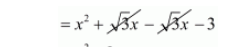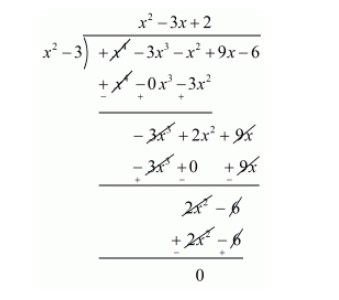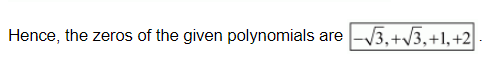# Obtain all zeros of the polynomial f(x) = x4 − 3x3 − x2 + 9x − 6,

Question:

Obtain all zeros of the polynomial $1(x)=x^{4}-3 x^{3}-x^{2}+9 x-6$, if two of its zeros are $-\sqrt{3}$ and $\sqrt{3}$

Solution:

We know that if $x=\alpha$ is a zero of a polynomial, then $(x-\alpha)$ is a factor of $f(x)$.

Since $-\sqrt{3}$ and $\sqrt{3}$ are zeros of $f(x)$.

Therefore

$(x+\sqrt{3})(x-\sqrt{3})=x^{2}+\sqrt{3} x-\sqrt{3} x-3$$=x^{2}-3$

$x^{2}-3$ is a factor of $f(x)$. Now, we divide $f(x)=x^{4}-3 x^{3}-x^{2}+9 x-6$ by $g(x)=x^{2}-3$ to find the other zeros of $f(x)$.By using division algorithm we have, $f(x)=g(x) \times q(x)+r(x)$.

$x^{4}-3 x^{3}-x^{2}+9 x-6=\left(x^{2}-3\right)\left(x^{2}-3 x+2\right)+0$

$x^{4}-3 x^{3}-x^{2}+9 x-6=\left(x^{2}-3\right)\left(x^{2}-2 x-1 x+2\right)$

$x^{4}-3 x^{3}-x^{2}+9 x-6=\left(x^{2}-3\right)[x(x-2)-1(x-2)]$

$x^{4}-3 x^{3}-x^{2}+9 x-6=\left(x^{2}-3\right)[(x-1)(x-2)]$

$x^{4}-3 x^{3}-x^{2}+9 x-6=(x-\sqrt{3})(x+\sqrt{3})(x-1)(x-2)$